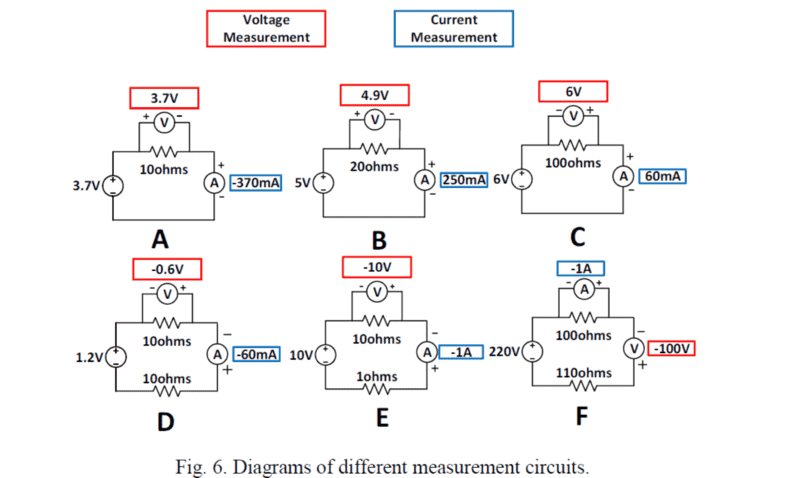# Which circuit shows the correct connection and values?

jaximus

## Homework Statement

Question:
For the circuits shown in Fig. 6, voltage meters and ampere meters are used to measure
the voltage across the load and their current flow. If the Red Probe is plugged into the “+”
terminal of the voltage meter and ampere meter, which circuit(s) show both the correct
connections and measurement values? State all correct answers.I= V/R

## The Attempt at a Solution

I have figured out that circuit D is correct.
Circuit F is wrong due to incorrect method of connecting the voltmeter and ammeter.
Circuit A and E has wrong polarities in one of their values.
Now Circuits B and D are tricky for me. Circuit B's voltmeter has a reading which is only 0.1 V lower than the EMF. Is this still considered correct?
As for Circuit D, The value of current I got through calculation was -0.91 A. Ammeter, however, shows a reading of -1 A. I am confused on whether or not this -1 A value is a rounded off answer. Can somebody please guide me on these 2 circuits.

#### Attachments

Gold Member
2021 Award
Circuit F is wrong due to incorrect method of connecting the voltmeter and ammeter.
correct
Circuit A and E has wrong polarities in one of their values.
That's not the only thing wrong with E, but yes they are both wrong
Now Circuits B and D are tricky for me. Circuit B's voltmeter has a reading which is only 0.1 V lower than the EMF. Is this still considered correct?
No, why would it be? These are obviously intended as ideal circuits so what you see is what you get.
As for Circuit D, The value of current I got through calculation was -0.91 A. Ammeter, however, shows a reading of -1 A. I am confused on whether or not this -1 A value is a rounded off answer. Can somebody please guide me on these 2 circuits.
It appears that you are talking here about E, not D.

Staff Emeritus
As for Circuit D, The value of current I got through calculation was -0.91 A
If current is -0.91A then a meter reading of -1A could not be considered acceptable. I'd say.

Gold Member
2021 Award
Calculate again what current flows in the last circuit, F.
Check out how the meters are hooked up (ignore the numbers)

•NascentOxygen
jaximus
correct
That's not the only thing wrong with E, but yes they are both wrong
No, why would it be? These are obviously intended as ideal circuits so what you see is what you get.
It appears that you are talking here about E, not D.